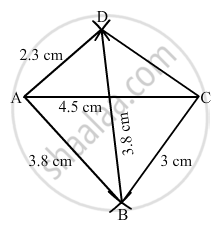Share

# Construct a Quadrilateral Abcd in Which Ab = 3.8 Cm, Bc = 3.0 Cm, Ad = 2.3 Cm, Ac = 4.5 Cm and Bd = 3.8 Cm. - Mathematics

Course
ConceptConstructing a Quadrilateral When Two Adjacent Sides and Three Angles Are Known

#### Question

Construct a quadrilateral ABCD in which AB = 3.8 cm, BC = 3.0 cm, AD = 2.3 cm, AC = 4.5 cm and BD = 3.8 cm.

#### Solution

$\text { Steps of construction: }$

$\text { Step I: Draw AC = 6 cm }.$

$\text { Step II: With A as the centre and radius 3 . 8 cm, draw an arc } .$

$\text { Step III : With C as the centre and radius 3 . 0 cm, draw an arc to intersect the arc drawn in Step II at B }.$

$\text { Step IV: With B as the centre and radius 3 . 8 cm, draw an arc on the other side of AC }.$

$\text { Step V : With A as the centre and radius 2 . 3 cm, draw an arc to intersect the arc drawn in Step IV at D }.$

$\text { Step VI: Join BA, DA, BC and CD to obtained the required quadrilateral }.$Is there an error in this question or solution?

#### APPEARS IN

RD Sharma Solution for Mathematics for Class 8 by R D Sharma (2019-2020 Session) (2017 to Current)
Chapter 18: Practical Geometry (Constructions)
Ex. 18.2 | Q: 1 | Page no. 6
Solution Construct a Quadrilateral Abcd in Which Ab = 3.8 Cm, Bc = 3.0 Cm, Ad = 2.3 Cm, Ac = 4.5 Cm and Bd = 3.8 Cm. Concept: Constructing a Quadrilateral - When Two Adjacent Sides and Three Angles Are Known.
S